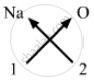# Work Out the Formulae for the Following Compounds : Sodium Oxide - Science

Short Note

Work out the formulae for the following compounds :

Sodium oxide

#### Solution

Valency of Sodium = 1
Valency of Oxygen = 2Formula for sodium oxide can be worked out by crossing the valencies of the constituent elements.

Therefore, formula for sodium oxide is: Na2O.

Concept: Laws of Chemical Combination - Law of Constant Proportions or Law of Definite Proportions
Is there an error in this question or solution?

#### APPEARS IN

Lakhmir Singh Class 9 Chemistry - Science Part 2
Chapter 3 Atoms and Molecules
Short Answers | Q 20.1 | Page 151